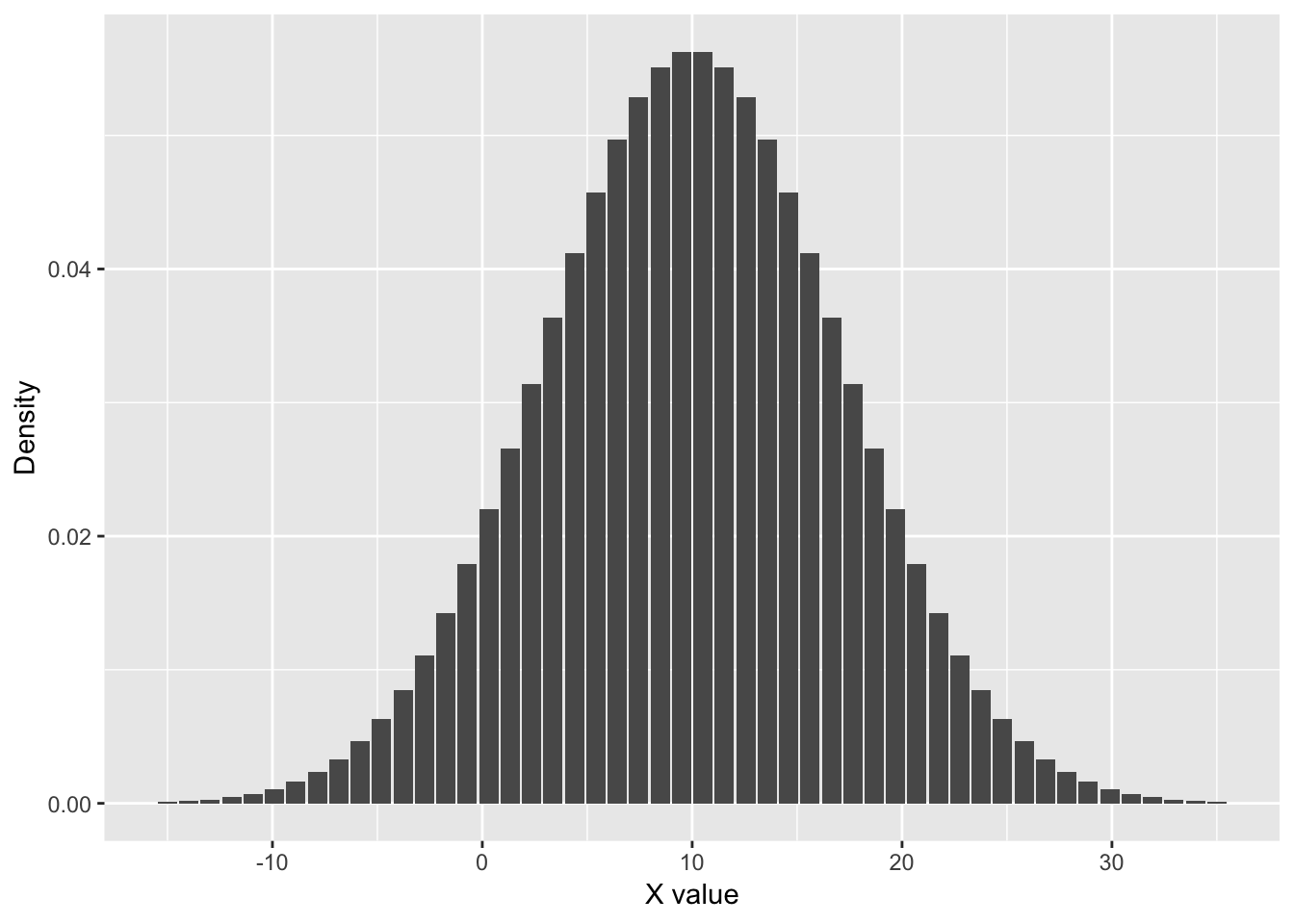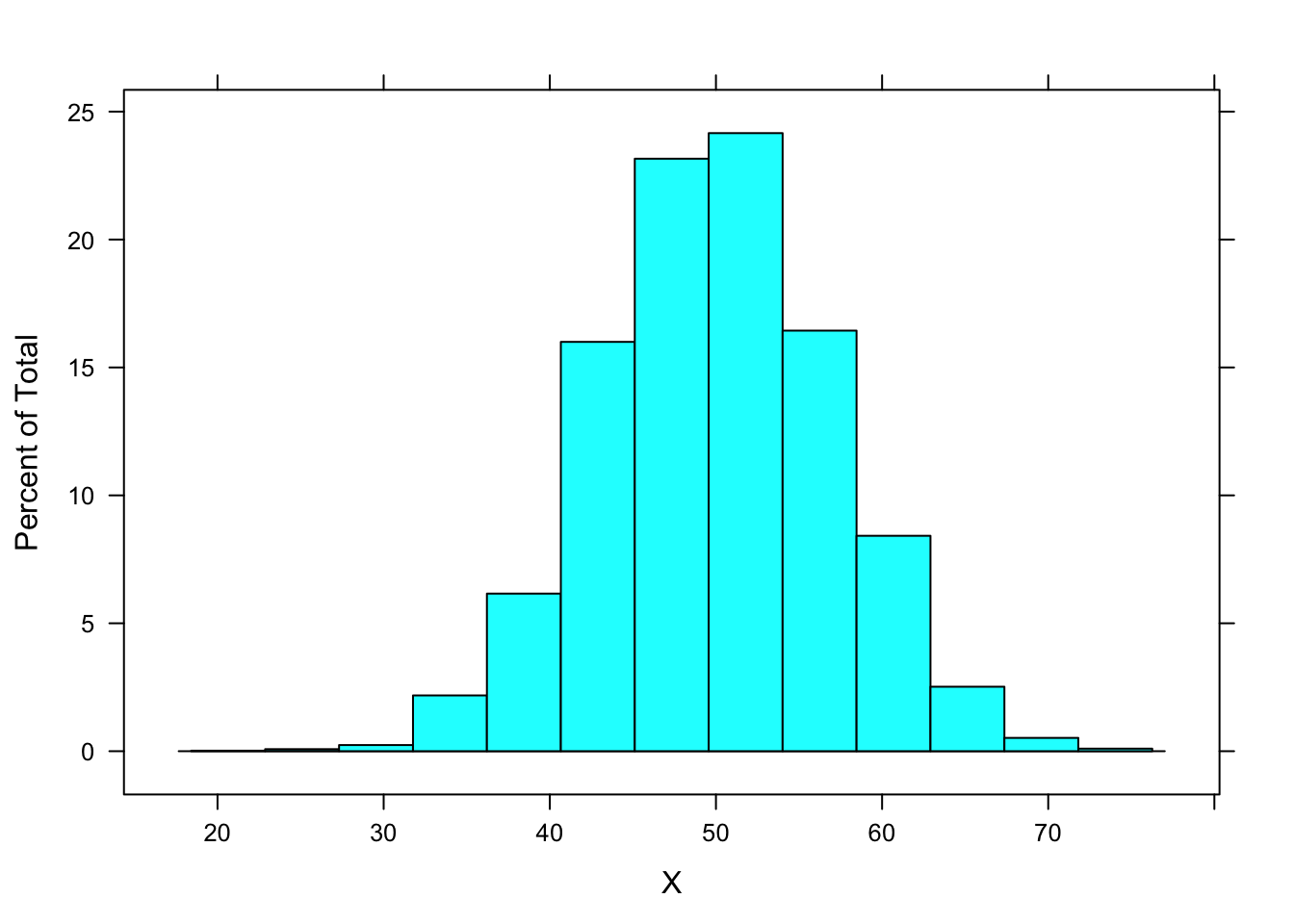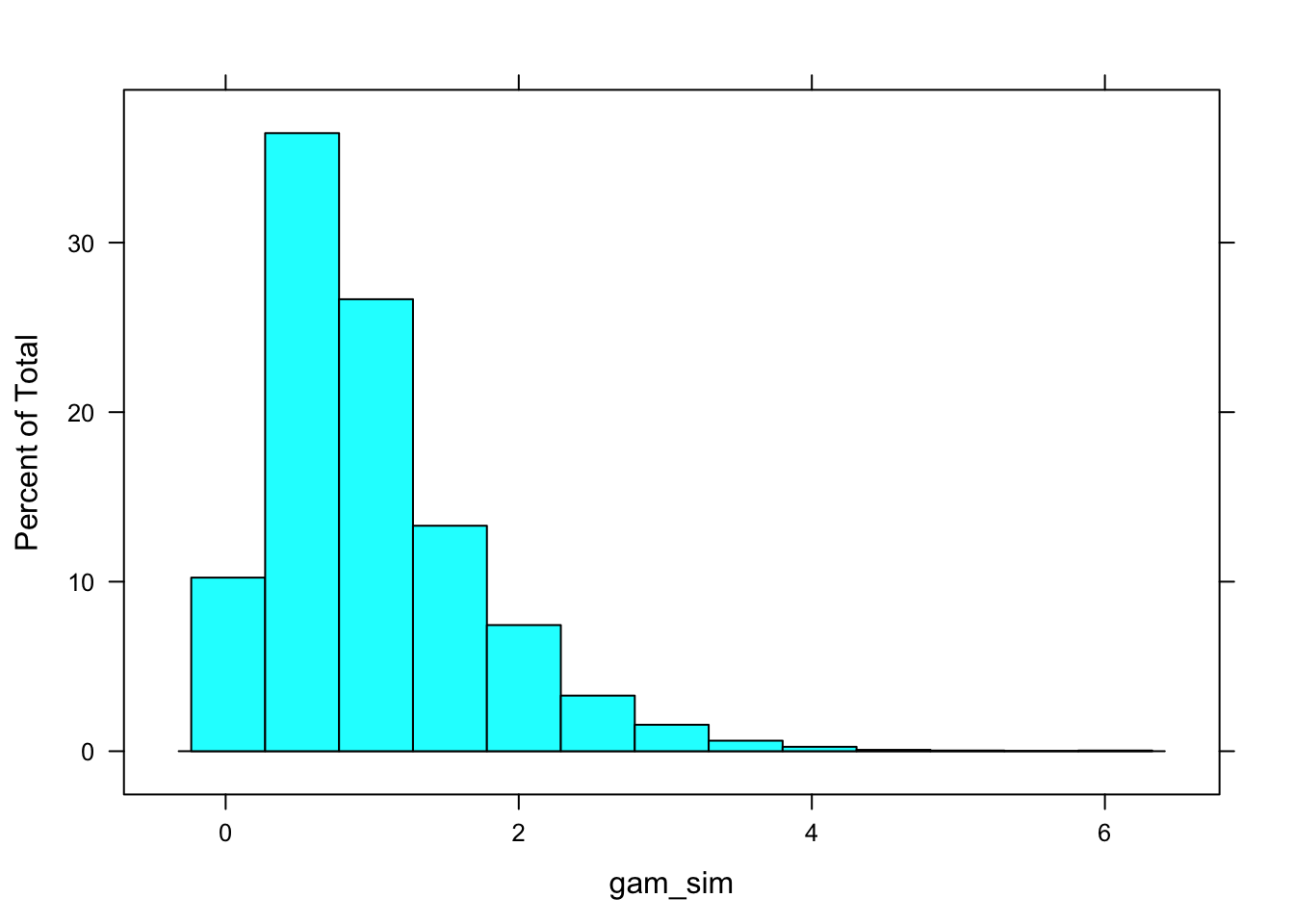# 1 Overview

Maximum likelihood estimation depends on choosing an underlying statistical distribution from which the sample data should be drawn. That is, our expectation of what the data should look like depends in part on a statistical distribution whose parameters govern its shape. The most common parameters for distributions govern location (aka ‘expectation’, often the mean) and the scale (aka ‘dispersion’, often variance). There are many different statistical distributions such as Gaussian (aka normal), Poisson, Gamma, and Beta. Crucially, probability distributions have parameter that define their shape and numerical properties.

In most of SEM, and regression for that matter, scientists often select the Gaussian distribution based on a belief that observed values tend to have a central tendency and symmetric dispersion around the tendency. Indeed, the central limit theorem posits that if a set of independent random variables is summed, the distribution approaches a normal distribution even if each variable is not Gaussian. Thus, if we imagine that the observed scores on a variable $$X$$ reflect the summed contribution of many unknown latent processes, a Gaussian assumption on the resulting distribution is often quite principled and parsimonious.

Let’s focus on the univariate Gaussian distribution as a test case for thinking about parameter fitting and likelihood methods. A Gaussian distribution has a mean and standard deviation that define the location and scale, respectively, of the data it describes. This is typically denoted:

$X \sim \mathcal{N}(\mu, \sigma^2)$ where X is a random variable distributed as normal with mean $$\mu$$ and variance $$\sigma^2$$. The parameters that control the shape of the distribution are sometimes called sufficient statistics (after Fisher) because if we know the values for these parameters, we can fully describe the distribution. That is, if the data are truly normal, then knowing the mean and variance alone is sufficient to have a detailed sense of how the scores will be distributed in a sample. For example, here is the density of normally distributed numbers with $$\mu = 10$$ and $$\sigma^2 = 50$$.

x <- seq(-15, 35, length=50)
hx <- dnorm(x, mean=10, sd=sqrt(50))
df <- data.frame(x=x, hx=hx)
ggplot(df, aes(x=x, y=hx)) + geom_bar(stat="identity") + xlab("X value") + ylab("Density")Importantly, when we are trying to identify the underlying parameters of a distribution such as the normal, we might try a fitting procedure (similar to OLS in regression). That is, we could try to minimize the discrepancy between the observed scores $$X$$ and the predicted data from a Gaussian distribution with mean $$\mu$$ and variance $$\sigma^2$$. By trying different values for the mean and variance, we could identify the values that best characterize the data. This is conceptually identical to trying to identify a best-fitting univariate regression line where we might test many values of the slope $$\beta$$. We could also posit that the data are drawn from some other population distribution, such as a Beta distribution, which would mean that we would need to adjust both the model and the parameter estimates.

# 2 Fitting a univariate distribution using maximum likelihood

Distribution fitting can be accomplished using a form of maximum likelihood estimation. Glossing over the details for now, imagine that we had a dataset of 5000 observations that have the following density:

X <- rnorm(5000, mean=50, sd=sqrt(50))
lattice::histogram(X)## 2.1 Statement of the problem

Given these observations, when we think about distribution fitting, we can ask the following questions. If we handed this dataset to a friend without any further information, could they figure out: a) the statistical distribution of the data, and b) the best-fitting parameters of that distribution? Stated differently, what is the likelihood that these observations are drawn from a candidate population distribution with its corresponding parameters. For example, what is the likelihood that these observations were sampled from a Normal distribution with $$\mu = 3$$ and $$\sigma^2 = 5$$?

## 2.2 Sample likelihood function

Answering the questions about the distribution and parameters of the distribution questions both turn out to be related to maximum likelihood estimation. That is, the question of maximum likelihood is to identify a set of parameters, $$\boldsymbol{\hat{\theta}}$$, that maximizes the probability of the data given the parameters. This is called the sample likelihood function:

$\mathcal{L}(D | \boldsymbol{\hat{\theta}}, \textrm{model})$

That is, what is the likelihood of the data, $$D$$, given a set of estimated parameters $$\boldsymbol{\hat{\theta}}$$ and a statistical model? The sample likelihood reflects the probability of the observed data given the parameters and model:

$\mathcal{L}(D|\boldsymbol{\hat{\theta}}, \textrm{model}) = P(D|\boldsymbol{\hat{\theta}}, \textrm{model}) = \\ P(\mathbf{d}_1, \mathbf{d}_2, ..., \mathbf{d}_n) = \prod_{n=1}^{N}P(\mathbf{d}_n|\boldsymbol{\hat{\theta}})$

where $$\mathbf{d}_n$$ denotes the vector of data for the nth individual and $$\prod$$ is the product operator (i.e., we multiply the probabilities for each subject together). Conceptually, this gives us the joint probability of all observations in the dataset, which is what we hope to maximize. Recall from basic probability theory that the joint probability of two independent events occurring simultaneously is equal to their product. So, if we ask “what is the probability that two coins flipped at once will both be heads?,” the answer is $$0.5 \cdot 0.5 = 0.25$$. Likewise, here, we are taking the product of the individual likelihoods under a candidate set of parameters $$\boldsymbol{\hat{\theta}}$$ to determine the joint probability of the observed data in the sample.

Because the product of many probabilities becomes very, very small, we typically maximize the log-likelihood to keep the computer on the rails (since maintaining numerical precision on infinitesimally small numbers is hard to do).

Returning to our simulated data, we could try to compute the parameters of different candidate distributions and compare their log-likelihoods in order to compare the relative fits.

## 2.3 Mis-fitting the data with the wrong distribution

Spoiler alert: for this dataset, we simulated 5000 observations from a normal distribution with $$\mu = 50$$ and $$\sigma^2 = 50$$. Thus, the groundtruth model is a normal distribution with these parameters. In empirical data, however, we essentially never have such details and instead have to infer the statistical distribution based on the data themselves.

Let’s start with the gamma distribution as one candidate that could describe these data. We know (because of our omniscient status on this problem) that this distribution is wrong in the sense that the data were not generated from gamma. Nevertheless, it could be plausible and fit well. The parameters of a gamma distribution are the shape, $$\alpha$$, and rate, $$\beta$$. For example, here is the shape of a gamma distribution with $$\alpha=2$$ and $$\beta=2$$:

gam_sim <- rgamma(5000, shape = 2, rate = 2)
lattice::histogram(gam_sim)Let’s take our dataset and ask the question, “What would be the parameters of a gamma distribution that would maximize the likelihood of these data?” Said differently, if we try to approximate the data with a gamma distribution, what parameters would yield the closest fit to the sample: Here is the estimate of the parameters of a gamma distribution that best fit the 5000 observed scores.

gfit <- suppressWarnings(fitdistr(X, "gamma"))
gfit$estimate ## shape rate ## 49.46 0.99 These are the best-fitting parameters of a gamma distribution that maximize the likelihood of the observed data. And you can see that they provide a reasonable fit to the data by simulating new data at those parameters. By eye, the plot below looks quite similar to a normal distribution with a mean of 50 and variance of 50 (i.e., SD ~7). newdat <- rgamma(5000, shape=gfit$estimate["shape"], rate=gfit\$estimate["rate"])
lattice::histogram(newdat)